Category Archives: Intermediate

Intermediate level information

Goal Seek in Excel

Formulas and Functions give you answers based upon input. If you know what a formula’s answer should be, but can’t derive the input value, Goal Seek is the tool for you.

I have a simple formula from my last post that takes one input, a Date in “m/d/yyyy” format, and returns the Day of the Year. This will tell me that Valentine’s Day — 14 Feb — is the 45th day of the year, or my birthday is day 311 this year.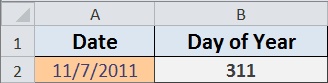The quickest way to find out what Date the 250th day of the year falls on is to use Goal Seek. For this example, here are the steps to take.

• Choose Data > What-If-Analysis > Goal Seek…
• In Excel for Mac and Excel 2003 choose Tools > Goal Seek…
• Click the Set cell box and select cell B2, which has the formula =A2-DATE(YEAR(A2),1,0)
• Click the To value box and type in 250, which is the outcome I want
• Click the By changing cell box and select cell A2, the value that Goal Seek will change
• Click OK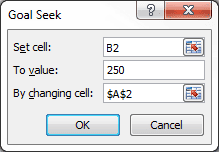Goal Seek searches for a solution and displays the following results.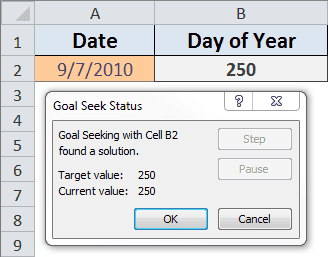Click OK to accept the changes, or click Cancel to restore the original value and leave the spreadsheet unchanged.

Note: Goal Seek works only with one variable input value.

Check out Goal Seek next time you know what a formula should be, but don’t have the time, inclination or smarts to figure it out.

What Day of the Year is It?

Given the Date in this spreadsheet, a simple formula using the DATE Function will return the Day of Year. Go ahead and type a Date value in cell A2 to see how the formula works, the spreadsheet is embedded from my SkyDrive. (Date format is US, “m/d/yyyy”)

The Day of Year Formula

The formula in cell B2 is:

= A2-DATE(YEAR(A2),1,0)

The DATE Function takes the Year from the Date you type in cell A2, and uses the zero (0) day of month 1 (January), which is the last day of the previous month. (I explained this in an earlier post).

The formula takes the Date you enter, and subtracts December 31st of the previous year to come up with the Day of Year.

You can copy the formula by double clicking cell B2, use Ctrl+A to select all the contents, and Ctrl+C to copy to the clipboard.

[UPDATE] Problems with SkyDrive

Apparently there are some problems with using the embedded worksheet on this web page. I added a public link to the file on Windows SkyDrive, but that seems to have some issues, depending on whether you use a Mac or Windows computer.

If you have problems with the embedded worksheet on this webpage, or with the web version on SkyDrive let me know in the comments what problem(s) you’re having, what hardware (Windows computer, Mac computer, iOS device – iPad, or other device) and what web browser (Firefox, Chrome, Safari, Opera, IE) you’re using. Thanks so much.

How Many Years, Months and Days Has it Been?

The DATEDIF Function comes in handy when you want to know how many years, months, and days there are between two dates. And even though it may be slightly flawed, it still beats the alternatives.

The following spreadsheet works well for birthdays or anniversaries. The DATEDIF Function is being used to generate the Years, Months, and Days.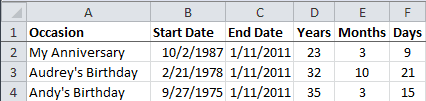The End Date column has the formula =TODAY().

The Years heading in cell D2 has the custom format ;;;”Years” which shows the text “Years” in the spreadsheet cell while hiding the underlying value “y” that you can see in the formula bar.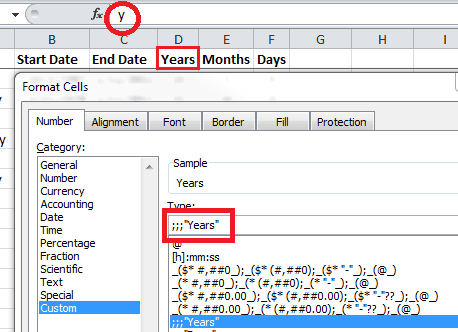The headings for Years, Months, and Days all have a custom format. The Underlying Value is what’s important for the next section.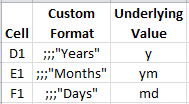Using the DATEDIF Function

The DATEDIF function is present in all current versions of Excel, but is only documented in Excel 2000. It was provided for compatibility with Lotus 1-2-3.

The Syntax is:

DATEDIF(start_date, end_date, unit)

Start Date and End Date are self-explanatory, although the end date must come after start date. The Unit is the type of information you want to return.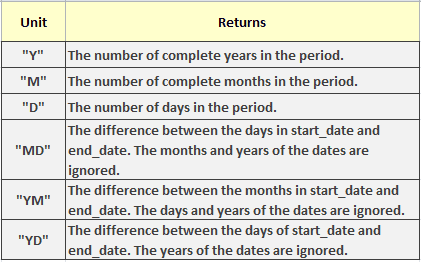The formula in cell D2 is =DATEDIF(\$B2,\$C2,D\$1) where \$B2 is the Start Date, \$C2 is the End Date, and D\$1 is the Unit. This gives you =DATEDIF(“10/2/1987”, “1/11/2011”, “y”) and returns 23 years.In the Months column I’m using the unit argument “ym” to return the number of months. (That’s the value in cell D1 you can’t see in the spreadsheet). The unit argument is “md” for the Days column.  The formulas in row 2 are:

• Years =DATEDIF(\$B2,\$C2,D\$1) = DATEDIF(“10/2/1987″,”1/11/2011″,”y”)
• Months =DATEDIF(\$B2,\$C2,E\$1) = DATEDIF(“2/21/1978″,”1/11/2011″,”ym”)
• Days =DATEDIF(\$B2,\$C2,F\$1) = DATEDIF(“9/27/1975″,”1/11/2011″,”md”)

A Problem with DATEDIF Function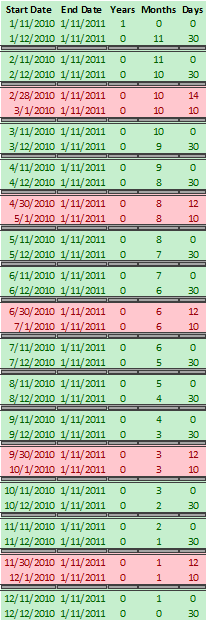I used a column of Start Dates for the past year and happened to notice a pattern with the Days column. Every month in the DATEDIF Function seems to have 31 days.

The 31 Day Cycle

The first pair of dates are the 11th and 12th of 2010. The first date is 1 year, zero months and zero days from 1/11/2011. The second date is zero years, 11 months and 30 days from 1/11/2011.

The green pairs show the Days cycle from zero to 30 days, which means a 31 day cycle.

Missing Days

Now look at the red pairs of data. These are the only non-sequential Days returned by the DATEDIF for the past year.

The first pair has start dates of 2/28/2010 and 3/1/2010. The first date is zero years, 10 months and 14 days from 1/11/2011. The second date is zero years, 10 months, and 10 days from 1/11/2011.

There are 3 days missing from this one day sequential difference.

The rest of the red pairs of data skip one day, which is the day after a month with less than 31 days.

For the spreadsheet I’ve put together this DATEDIF anomaly is not going to make me lose any sleep. Missing a day or two in 10 to 50 years is not very bothersome.

XLSX Format (Excel 2011, 2010, 2007, 2008)

XLS Format (Excel 2003, 2002, 2000)

The Repeat Command in Excel for Mac

Adding the Repeat command icon to the Quick Access Toolbar in Excel for Windows is a simple process that I explained in a blog post last week. Excel for Mac — 2011 or 2008 — doesn’t have a Quick Access Toolbar, but you can customize the toolbars and menus.

Add the Repeat Icon to the Standard Toolbar

• Right-click the toolbar and select Customize Toolbars and Menus…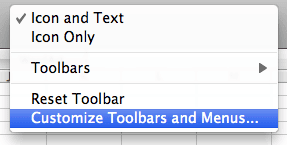• At the top of the dialog box select Toolbars and Menus
• Select the Show checkbox for the Worksheet Menu Bar
• In the Worksheet Menu Bar click Edit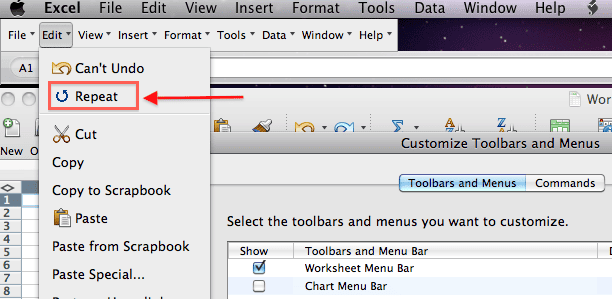• Select Repeat and while holding down the Control key, drag to the Standard Toolbar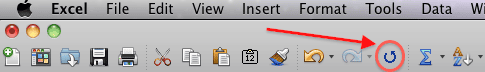• Click OK

Strangely enough, if you select Commands at the top of the Customize Toolbars and Menus dialog box, then select Edit from the Categories window, there is no Repeat command in the commands window. This is why I thought it didn’t exist in Excel for Mac.

The Three Different States of the Repeat Icon

The Repeat icon becomes active and has color when the current command can be repeated. It will be grayed-out if the current command can’t be repeated. After there’s been an Undo action, the Repeat icon will change to a Redo icon.

Turn on Toolbar ScreenTips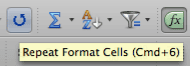There’s an option to show shortcut keys in the toolbar screen tips that’s nice to have turned on. It shows the keyboard shortcut for the Repeat command to be Cmd+6.

• Right click the Standard Toolbar
• Select Customize Toolbars and Menus…
• Click the Show shortcut keys in ScreenTip checkbox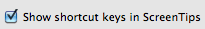• Click OK

Redo and Repeat Commands in Excel [Updated]

I always thought the F4 key was used to Repeat the last command, and the keyboard shortcut Ctrl+Y was used to activate the Redo command. I don’t use Redo very much, but the keyboard shortcut Ctrl+Z for the Undo command is one of my favorites. Toolbar icons for Undo and Redo command are common, yet I found there’s a toolbar icon for the Repeat command (Windows only).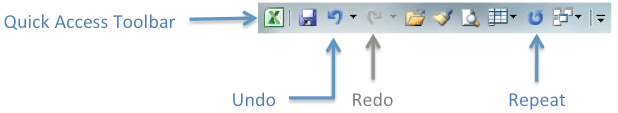Adding the Repeat icon to the Quick Access Toolbar is a simple way to distinguish what commands can be repeated. The Repeat icon will be grayed-out if the current command can’t be repeated. It becomes active and has color (as shown above) when the current command can be repeated. After there’s been an Undo action, the Repeat icon will change to a Redo icon.

• Select Popular Commands in the left-pane drop-down list (All Commands works too)
• Click inside the left-pane, press the R key, scroll down and select Repeat, then click Add
• In the right-pane move the Repeat icon up to the position you want
• Click OK

Excel: Repeat Last Action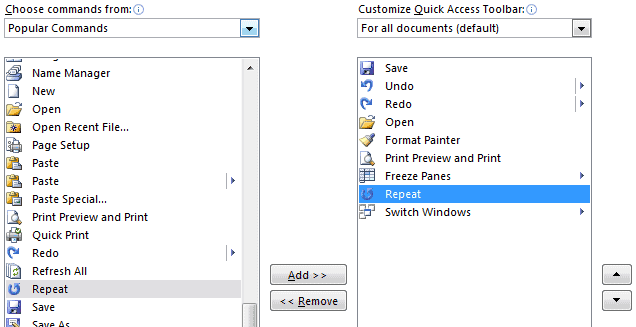When you perform or use a command in Excel to repeat your last action that’s compatible with the Repeat command, its icon on the Quick Access Toolbar will be colored, as shown below. A very good way to know if the Ctrl+Y or F4 shortcut will work.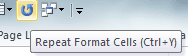The exception is, that after you use the Ctrl+Z shortcut or the Undo command icon, the Repeat icon will change to be colored AND look like the Redo icon.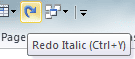Example: I like some of my data table headings to be Bold and Centered. After doing so in the Format Cells dialog box, the Repeat command (F4 or Ctrl+Y) will apply those changes to other cells.

Install the Repeat icon on the Quick Access Toolbar and play around with it. You may discover a handy use for the Repeat command.

Excel for Mac [UPDATED]

The Repeat icon doesn’t does exist in Excel for Mac, but it will require a separate post to explain. However, you can change the F4 button to act like the Windows version.

Fixing the F4 Button in Excel for Mac

The F4 key in Excel for Windows is normally a shortcut key to Repeat the last command. In Excel for Mac the F4 key is assigned to the Paste command, which is driving me crazy so I decided to do something about it.

Default Excel F4 Keyboard Assignment in Excel for Mac

There are four keyboard shortcuts assigned to the Paste command in Excel for Mac — Shift+Del, Control+V, F4, and Command+V.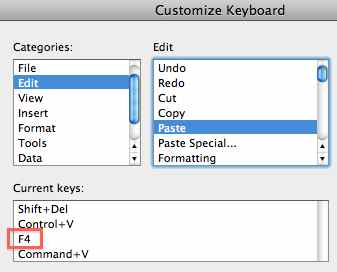Overkill, don’t you think? So I’m thinking the F4 button can be reassigned with no problems, unless, of course, you’re in the habit of using the F4 key for the Paste command.

Reassign the F4 button in Excel for Mac

• Select the Tools menu, and click Customize Keyboard…
• In the Categories: box select Edit
• In the Edit box, select Redo
• Select the Press new shortcut key: box
• Press the keyboard combination fn+F4 key (or just F4 key if you’ve setup the Mac function keys normally)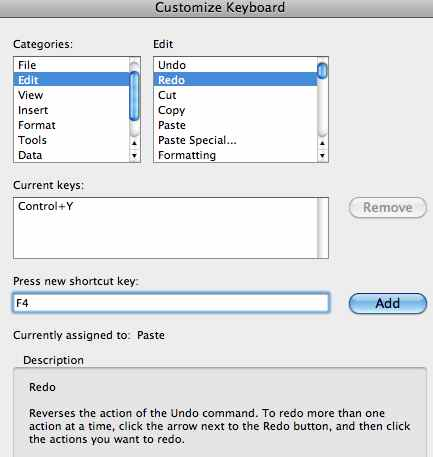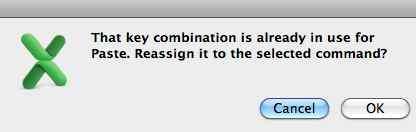• Click OK to reassign the Paste command to the Redo command.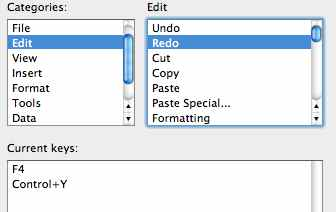The Excel F4 button will now work with the Redo command, which is similar to how Excel for Windows is setup.

Note: You have to make this change to each version of Excel for Mac. For instance the above procedure was done in Excel 2011, but my Excel 2008 version of Excel for Mac wasn’t affected and has to be done separately.

The TRIM Function in Excel and VBA

Excel’s TRIM Function is a great way to remove extra spaces before, after, and within text in a spreadsheet. However, if you think the identically named VBA function does the same thing you’d be sadly mistaken, as I was once upon a time.

Here’s a normal sentence.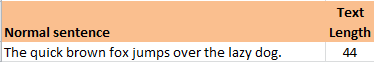Here’s a sentence with extra spaces – two before, one after, and several within the sentence.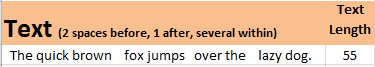Here’s a comparison of the two TRIM Functions.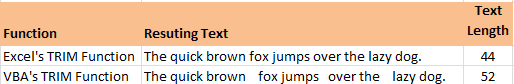The Excel TRIM function reemoves all spaces from text except for single spaces between words.

The VBA TRIM Function only removes leading and trailing spaces.

The VBA TRIM Function Warning

If you need to remove spaces within text and are using VBA Trim function, it’s best to use Application.WorksheetFunction.Trim(your text here) to get the job done.

Note: This is a public service message. 🙂

The VLOOKUP Function in Excel

VLOOKUP is an Excel Function that’s used to look up something. It takes four arguments. The first argument is the Something. The second and third arguments are the Look Up part. And the last argument is optional, but the most important, and is either TRUE or FALSE.

Technically the first argument is the lookup_value, but it’s just the Something we’re going to use in the Look Up part. The second and third arguments are the table that holds the look up data and column where the return value resides.

The VLOOKUP Function takes the Something and tries to find a match in the first column of the table.

Now here’s where the TRUE and FALSE part comes in. If the fourth argument is FALSE there will be an exact match. I use FALSE for virtually every VLOOKUP formula, so we’ll consider the TRUE option later.

So to continue, we have the Something lookup_value, and have found an exact match in the first column of the table. This also tells us the particular row of the table where our answer resides.

The third argument tells us the column number of the table to look in. Knowing the row and column gives us the cell where we can find the look up value.

Using VLOOKUP with FALSE as the Last Argument

So a quick review. We use the VLOOKUP function, where the first argument is something like “Banana.” The second argument tells us where the table is that’s holding the lookup data. We match the word “Banana” in the first column of the table, then slide along the same row to the column number that’s given by the third argument and locate the cell holding the lookup value, which is then returned to the cell where we put the VLOOKUP formula. And of course all of this is guided by the fact that the last argument is FALSE, which tells the VLOOKUP function to get an exact match to “Banana.” (Upper and lower case are considered equivalent.)

Let’s look at a simple example. I have a worksheet named “MyData” that has a table with values we want to look up, shown below.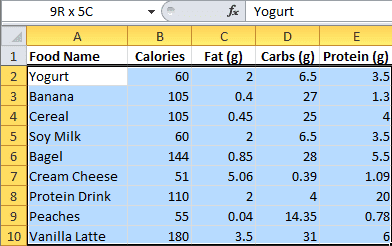Here’s a different worksheet with the VLOOKUP formula.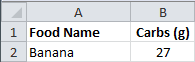Cell B2 holds the VLOOKUP formula:

=VLOOKUP(A2,MyData!\$A\$2:\$E\$10,4,FALSE)

The first argument is cell A2, which is “Banana.”  The second argument is the location of the data table on the MyData worksheet in the range A2:E10. The third argument is the number 4, because we want to look up Carbs (g) in the fourth column, and the last argument is FALSE to find an exact match.

Here’s the VLOOKUP dialog box that you can use in creating the formula. The picture rotates through the four different arguments — Lookup-Value, Table_array, Col_index_num, Range_lookup — so you can see the explanation for each.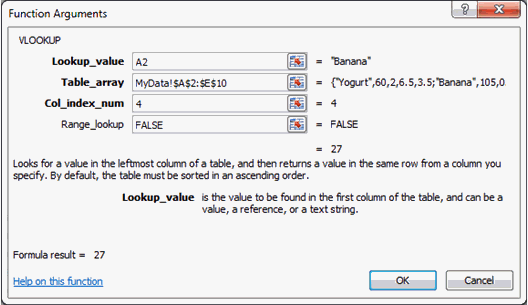One thing you need to know about using FALSE for the fourth argument is that, if there’s more than one of the Something, the VLOOKUP formula will only find the first one in the list.

Using VLOOKUP with TRUE as the Last Argument

Here’s what you need to know about using TRUE as the last argument.

1. The values in the first column must be placed in ascending order, otherwise you might not get the correct value.
2. If you omit the last argument, VLOOKUP considers it to be TRUE.
3. With TRUE, you get an exact or approximate match. If no exact match is found, then you get the next largest value that’s less than the lookup_value. (And after all these years, this still strains my brain to near the breaking point.)

Here’s a simple example that shows how grades are returned by a VLOOKUP formula when given some numerical scores.  The data table for grades is shown here.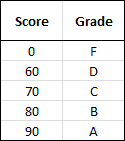• Any score with values from 0 – 59 will return an F grade.
• Any score with values 60 – 69 will return a D grade.
• Any score with values 70 – 79 will return a C grade.
• Any score with values 80 – 89 will return a B grade.
• Any score with values 90 – 100 will return an A grade.

I covered this in an earlier article: Grade Formulas in Excel.

Here’s the worksheet with Students, Scores, and the VLOOKUP formula.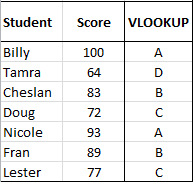And here’s the VLOOKUP formula used with TRUE as the fourth argument.

The first argument is the scores in the Score column, which I gave the name: Scores. The second argument is the table that I’ve named GradeLU. The third argument is 2 because the second column has the Grades.

If the first argument is 83, the VLOOKUP formula looks to the GradeLU table, and since the last argument is TRUE, it looks in the first column and matches the 80, then goes to the second column and returns the grade B.

VBA Help System Lacking in Excel 2011

Since never using the VBA Editor in Excel 2011 for Mac was a slight embarrassment, I thought to give it a whirl with a half-written function that was started in Excel 2010. I quickly found out the Editor’s windows have more of a free-floating style, but the programming looked to be quite the same.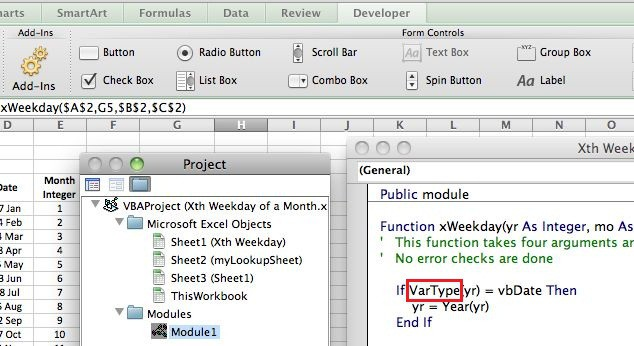Until I wanted to find out whether to use the TypeName or VarType function and tried to access Help. This is something they make sufficiently hard enough that I didn’t find either function definition before giving up and deciding to write about the futility of being dropped into a maze.

But first I switched back to Excel 2010, selected VarType in the VBA Editor and activated Help, which quickly generated a nice pop-up window with all the facts I needed about the VarType Function, as you can see below.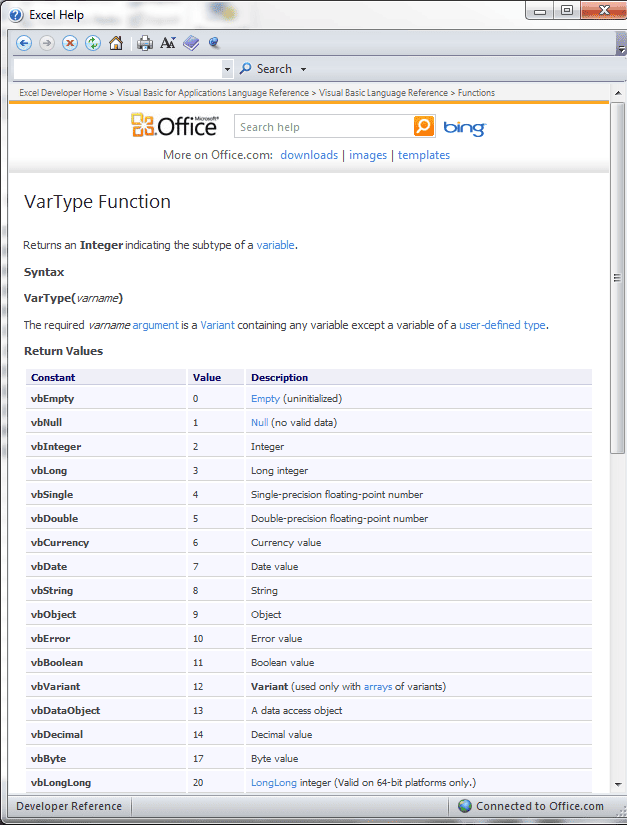When you try this in Excel 2011, the following screen is the entrance to the maze.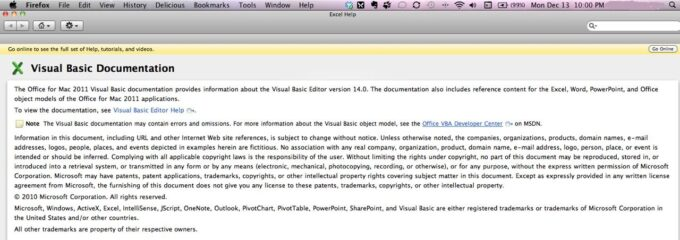click for larger image

Continuing on from this screen is when you start feeling that something’s all jumbled and confused in the Help system for VBA in Excel 2011. I don’t recommend going on any further unless you’re desperate.

Oh what a tangled web they weave.

Excel ISNUMBER or IsNumeric Function

I don’t like it when Excel worksheet functions are different from their VBA counterparts. Makes for some aggravation.

For example, I have a user generated data range that’s supposed to be made up of either numbers or empty cells. However, Excel users sometimes bump their keyboards inadvertently (when you hit the space bar) and things like a space character can get entered into an otherwise empty cell, and go undetected to the naked eye.

VBA Programming

As part of a VBA macro I looped through each cell in this data range and performed an operation for any cell value greater than zero. This was a good plan for a data range having only number values or empty cells. (Well, not really as you’ll see below.)

However, I not only came across a space character in the user data, but also an accent character (`). As you can see in third column of the the chart pictured below, (>0) greater than zero is TRUE for the space character and the accent mark.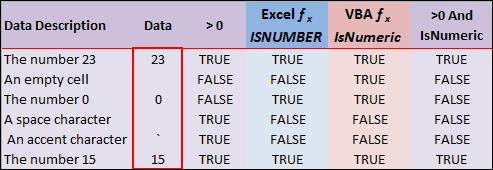What I really wanted was the Excel ISNUMBER fucntion that only shows TRUE when, well, there’s a number. (Fourth column)

So I tried the VBA Excel IsNumeric function in my macro, thinking it to be the same thing, and found that it didn’t work as I had imagined. For some reason the IsNumeric function shows TRUE for and empty cell as you can see in fifth column of the chart.

I wrote a function called IsNum() to simulate the VBA function IsNumeric in the worksheet.

Function IsNum(data As Variant)
If IsNumeric(data) Then
IsNum = True
Else
IsNum = False
End If
End Function

The difference seems to be that the Worksheet Function ISNUMBER takes a Value for an argument, and the VBA function IsNumeric takes an Expression for its argument.

My solution is to use both greater than zero (>0) AND IsNumeric for my logic gate to perform further operations. (Last column in the chart) So in looping through the data range above, operations would only be performed on the numbers (23 and 15).

Lessons Learned About Excel ISNUMBER or IsNumeric Function

1. Now I know that an accent character (`) is greater than zero.
2. The VBA function IsNumeric is different than the Excel worksheet function ISNUMBER.
3. Testing doesn’t always bring out all the ways a user can change the data. Only time and more users can do that.
4. One logical operation in a very large VBA macro (9 subroutines w/ 2 functions) can bring down the whole show.

Calculate Hours Between Two Dates and Times in Excel

Recently I was asked how to subtract time in Excel (time difference) or how to calculate the number of hours between two points in time on different days. Since this was in a reader comment, I gave a brief answer that requires a fuller account here.

Dates and Times are all part of the master plan in Excel. Once you “get” the fundamentals, the rest is just icing on the cake.

A Date value in Excel looks like this: 40519
A Time value in Excel looks like this: 0.58333

Cell formatting changes how you see these numbers.

The Date: 7 Dec, 2010
The Time: 2:00 PM

How to Calculate Time Between Dates in Excel or the Duration Between Two Dates

If you want to calculate time between dates in Excel or the duration between two dates, you need to understand what they mean first. When you type a Date into Excel, you may never see the underlying number, like 40519, but it’s there nonetheless. This a date serial number and it makes Date calculations easy.

You ask, “Why is this such a weird-looking number?” Well the Excel folks started a numbering system with Dates. In Excel for Windows they gave 1 Jan, 1900 the serial date number of 1, then continued numbering until this day and beyond. So serial number 40519 represents 7 Dec, 2010.

In Excel for Mac they started numbering Dates beginning with 2 Jan, 1904. (don’t ask) So the serial date 40519 represents 8 Dec, 2014 (Actually it’s known as the 1904 date system. To be clear, Macs can change Excel settings to use the 1900 date system).

How to Subtract Time in Excel (Time Difference)

When you type 2:00 PM into a cell in Excel the underlying value is a fraction, but Excel interprets this as a time serial number and formats the cell accordingly.

Try typing 0.25 into a blank cell, then change the cell formatting to a TIME format, and you’ll get something like 6:00 AM.

As an aside, you can calculate this fraction for any time value during the day by taking the total number of seconds that have passed from midnight until your time value and dividing by 86,400 seconds in a day.Dates and Times Together

In Excel the unit of time is “the Day,” a key fact to know. You’ll notice that Dates are integers, and Time is a fractional number. You can add the two together to get a Date/Time format.

So adding a Date serial number, like 40518, to a Time serial number, like 0.25, gives us 40518.25. Formatting the cell holding this value using “d mmm, yyyy h:mm AM/PM” will show 6 Dec, 2010 6:00 AM.

You can also enter something like 7 Dec, 2010 2:00 PM into a cell and Excel will recognize this as a Date/Time format. However, if you change the cell formatting to General, the underlying number is 40519.05833.

So hopefully by now you can see that subtracting two Date/Time formatted numbers can be done mathematically. Subtracting 6 Dec, 2010 6:00 AM from 7 Dec, 2010 2:00 PM is done by Excel “underneath the hood” as 40519.05833 – 40518.25 and the result is 1.3333.

Calculating Hours Between 2 Dates and Times

If we recall that the unit of time is “the Day,” this value represents 1-1/3 days of time.  Since there are 24 hours in a day, converting to hours is a simple multiplication 24 * 1.3333 = or 32 hours. (24 * 4/3 to be more precise)

Time Between Two Times / Dates

Finding the number of hours or the time between two times / dates is simple, just subtract the start date/time from the end date/time and multiply the result by 24 hours.

If you want to enter the dates and times separately (which is loads easier than typing in a date/time in one cell) then add the date/times together.

Hours = ((End_Date+End_Time)-(Start_Date+Start_Time))*24

Here’s a look at a typical worksheet designed to calculate the hours between two dates.As you can see, the formula for Hours, in cell F2, shows in the formula bar. And row 3 contains General formatting so you can view the date/time serial numbers for row 2.

Change the formatting for cells B2:E2 to match what you normally use for Date and Time data entry.

I hope this article helps you to better understand how to calculate time difference in Excel.

Microsoft Office 2011 Document Connection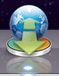One of the neat programs you get with Microsoft Office 2011 is Microsoft Document Connection. It allows you to interface with your SkyDrive without going through a web browser.

Once you open the program and sign in to your SkyDrive account all your online files are accessible. You can open the files in Read Only or Edit mode.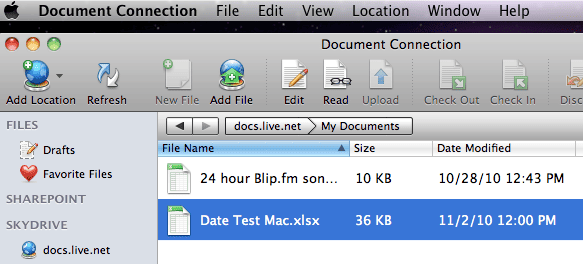In Preferences I set all my files to open in Edit mode so that when I double click on a file, Excel 2011 downloads, then opens the file so I can make changes.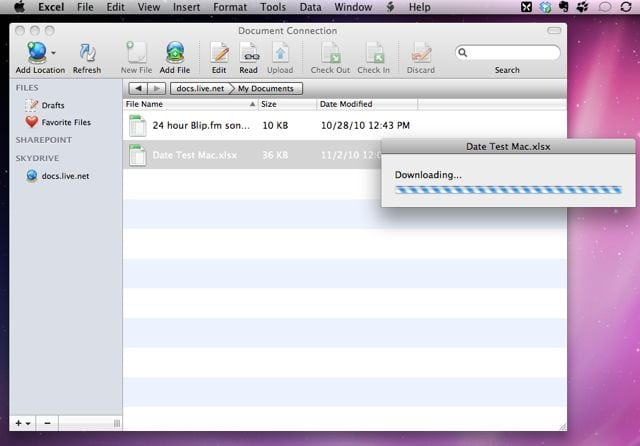There’s also an option to upload a file directly to SkyDrive. And you can also get a URL link to any file on your SkyDrive.

I don’t know if a similar program is available with the Windows version because I didn’t purchase the entire Office suite of programs (too expensive). With Excel 2010 I have to launch my web browser to connect to my SkyDrive account in order to get access to my cloud storage files.

Files in the cloud are much easier to access and work with using Microsoft Document Connection. Take advantage if you have a Mac.

Equation Editors in Excel 2010 and Word 2011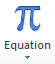The Equation Editor is new in Excel 2010 and designed to create a mathematical equation as a graphical object. I didn’t find it in Excel 2011 for Mac, but ran across an article showing they put it inside Word 2011. How very strange.

Here I’ll review the Excel 2010 version of Equation Editor first, then briefly compare Mathematical Equations in Google Docs, and come back to the Word 2011 version of Equation Editor.

Excel 2010 Equation Editor

The Equation Editor in Excel 2010 is difficult to navigate at first. The second time I tried creating an equation it got slightly easier, and the third time with the same equation (shown below) I seemed to almost get the hang of it.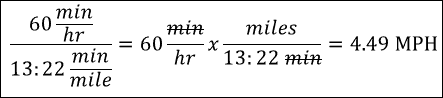The trick I learned was to just type the equation in and let the Editor do its thing in rearranging what you’re typing. On the Equation Tools Ribbon are two helpful selections: Professional and Linear (shown below). Playing around with these helped me understand how the Editor can manipulate simple equations.

Having said that, advanced equations will take some time to figure out, but this editor should handle most anything you can throw at it.

Entering an Equation

To enter an equation with the Equation Editor go to the Insert tab on the Ribbon, and click Equation. This gives you a text box with the words “Type equation here.” You’ll also notice two additional menu tabs on the Ribbon: Equation Tools, which becomes active, and Drawing Tools.

There are varied Symbols readily available for insertion on the Equation Tools Ribbon tab (shown above). And several drop-down menu items that expand to show even more symbols for things like: Fraction, Script, Radical, Integral, Large Operator, Bracket, Function, Accent, Limit and Log, Operator, and Matrix. (Shown below)

Clicking the Equation drop-down arrow will reveal several predefined equations that can be inserted as starting point for your equation. This how I started my first equation, which did me no favors. I fared much better just typing in the formula and letting the Equation Editor move things around.

One Annoyance

One of the problems with the Equation Editor is that if you stray to far outside the equation, yet still inside the text box, the Equation Tools tab disappears and you’re stuck with the Drawing Tools tab only. Maddening!

I looked at the new Mathematical Equations in Google Docs and it seemed easier to learn, but is not nearly as powerful as the Equation Editor. For example, the equation I created above was done in a Google Docs document with Mathematical Equations. I wasn’t able to do a strikethrough for the min text, like this: min.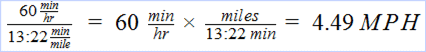And there wasn’t a good way to export this object out of Google Docs.

Equation Editor in Word 2011 for Mac

The Equation Editor in Word 2011 for Mac looks to be identical to the one in Excel 2010. You start an equation from the Document Elements tab on the Ribbon, by clicking Equation or the drop-down menu arrow and selecting a built-in equation.

The Equation Tools tab immediately becomes visible and active after starting an equation. I created the same formula with relative ease.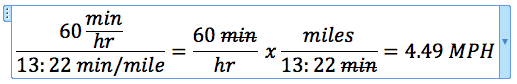I kind of like the feel of this Word 2011 version better than what’s in Excel 2010. Go figure.

Convert Pace to MPH and Back Again in Excel

Excel is a great tool for figuring stuff out, like for conversions that aren’t easy to do in your head. Here I’m converting Pace to MPH and then reversing the process, converting MPH to Pace, to create a conversion chart.

My Conversion Problem for Minutes per Mile to MPH

I track my Average Pace when out walking for exercise by using the iPhone App Walkmeter, then log that information into the Lose It App. The problem I have is converting my Average Pace to Miles per Hour (MPH).

Below you can see my average pace is 13:22 per mile, but Lose It wants me to pick from a list of MPH values.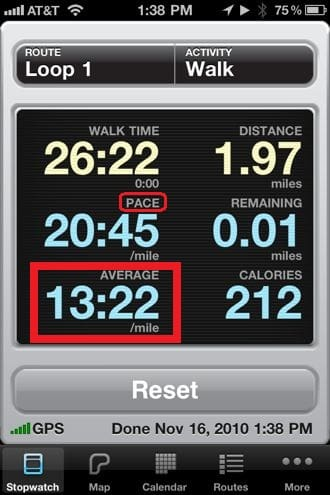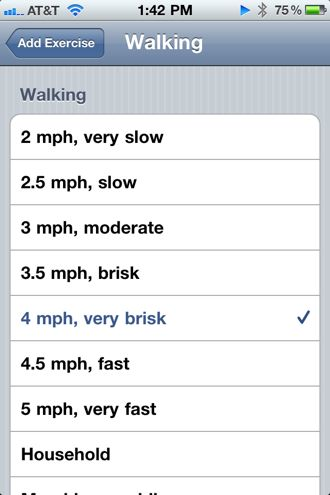Simple Conversion Equation

You can use algebra to work out how to convert Pace, in minutes per mile, to MPH.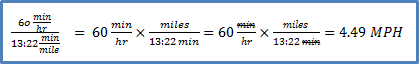The problem with this is that Walkmeter shows the Average Pace in Minutes and Seconds per mile, which is not decimal minutes per mile.

Converting Minutes and Seconds

There are a couple of ways to convert minutes:seconds to decimal minutes. The first mimics what I would do by using a calculator and the second is strictly an Excel thing to convert minutes per mile to MPH.

Decimal Minutes

Divide the seconds by 60 then add the result to the number of minutes to get decimal minutes.

B2 =MINUTE(A2)+SECOND(A2)/60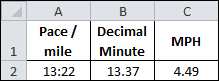This solution uses the Excel Functions MINUTE and SECOND.

Decimal Hours

Convert 13:22 to a time serial number by using the TIME Function, then multiply by 24 to get decimal hours.

B2 =TIME(,MINUTE(A2),SECOND(A2))*24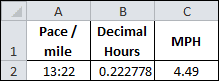The TIME Function above has three arguments:

• Hour, which is blank
• Minute, which uses the MINUTE Function
• Second, which uses the SECOND Function

Convert to MPH

Now that I’ve converted minutes and seconds to either decimal minutes or hours, converting Pace to MPH can be completed in a second step.

Using Decimal Minutes

Simply divide 60 min/hr by the 13.37 pace/mile to get 4.49 MPH.

C2 =60/B2Combining equations in cells B2 and C2 gets us the conversion in one big equation:

MPH =60/(MINUTE(A2)+SECOND(A2)/60)

Using Decimal Hours

Here we simply invert the decimal hours to get our answer, one (1) divided by 0.0222778 decimal hours, gives us 4.49 MPH.

C2 =1/B2Again, we can combine equations to get:

MPH =1/(TIME(,MINUTE(A2),SECOND(A2))*24)

MPH to Pace Conversion

For a given set of MPH values I want to convert to Pace per mile and show the result in a minutes:seconds format. Essentially reversing what I just did above.

The simplest way to do this is to realize that the time serial number is based on seconds. We’ll also use the fact that 1 hour = 3600 seconds.

When we divide 3600 by an MPH value, it gives us the number of seconds it takes to go one mile. Plugging these seconds into the TIME Function will give us our answer, but as a time serial number. We can then use a custom format of mm:ss for the Pace range and the conversion is complete. The equation for cell B2 is:

=TIME(,,3600/A2)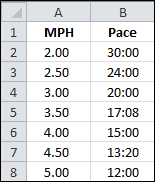This formula works because column B is formatted using the mm:ss custom format.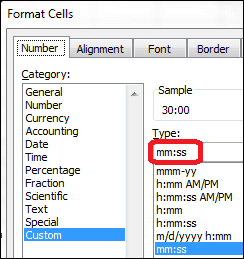So now I have a conversion chart for MPH to Pace and can probably remember that a pace of 13:22 is close to 4.5 MPH, which is helpful to me. How about you?

There are several ways to turn student scores into letter grades. I recently came across a nested IF formula that did the trick, but it seemed rather complicated.

A better solution would be to use a VLOOKUP formula with a Grade Lookup Table, but then it occurred to me that the VLOOKUP formula could stand alone by using an Array Constant.

For all these solutions I’m using the following grade scale:

• 0 – 59 = F
• 60 – 69 = D
• 70 – 79 = C
• 80 – 89 = B
• 90 – 100 = A

The Nested IF Solution

Using the scale above as a guide, the following nested IF formula will turn a score from 0 to 100 into the correct letter grade.

=IF(Score>=90,”A”,IF(Score>=80,”B”,IF(Score>=70,”C”,IF(Score>=60,”D”,”F”))))

This formula uses Score, which is a named range that contains all the student scores.

A VLOOKUP with a Grade Lookup Table

The following formula will also give the correct letter grades.

This formula has four arguments. Score refers to the student score that’s being looked up.

GradeLU is the Grade Lookup Table that’s on another worksheet and is a named range.

The two (2) means that a number from the second column will be returned from GradeLU.

The TRUE means the student score will be approximately matched to the first column from GradeLU. This is what allows a score of 72 to be matched to 70 and consequently return a letter grade of C.

A VLOOKUP with an Array Constant

The Grade Lookup Table can be replaced with an array constant. In the formula above, the second argument, GradeLU, could be replaced with the following:

{0,”F”;60,”D”;70,”C”;80,”B”;90,”A”}

However, instead of typing this into the formula each time, we can create a Named Constant in the Define Name dialog box shown below.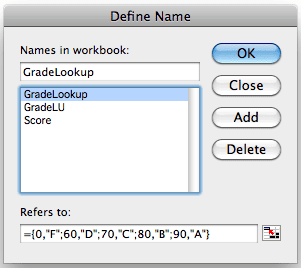By creating GradeLookup as a named constant array, the formula can be shortened to:

This formula does not need to reference a separate Grade Lookup Table on a worksheet because all the values are located in the named array constant, GradeLookup, which is now located in Excel’s internal memory.

Create a Named Constant Array

Bring up the Define Name dialog box and type in the name GradeLookup. Then delete the contents in the Refers to: text box and type in {0,”F”;60,”D”;70,”C”;80,”B”;90,”A”} exactly and click OK. Excel will add the equals sign (=).

How you access the Define Name dialog box depends on the version of Excel your using:

• In Excel 2003, and Excel 2008 and 2011 for Mac, use the menu selection Insert-Name-Define…
• In Excel 2007 and 2010 click the Ribbon tab Formulas and select Define Name.